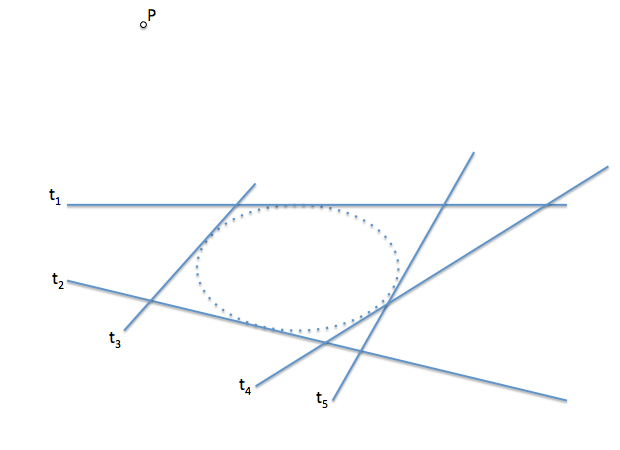# Geometría proyectiva: Tangent from a point to a conicalThe defining the conical projective lets start solving classic problems of identification of new elements of the conical (new points and tangents in them), and find the intersection with a line or a tangent from an external point. These problems can be solved by various more or less complex methods and conceptually more or less laborious paths.

We have seen how determine the points of intersection of a straight line with a conical defined by five points. We will then see the dual problem.

This problem consists of determining the possible two lines from a point to a Conic defined by five tangent-tangent.

As an auxiliary tool to use a resolution circumference as a series of second order.The problem determined by five tangent (t1 … T5) and a point P. The conical not find mapped. The analysis is shown to serve as conceptual support but we can not use the curve directly in solving the problem.If we understand the Conic as an envelope curve of tangent lines, in particular, the straight lines that projected pairs of homologous points of two projective series and it is tangent to the bases of these series, We can generate two series with two bases of the tangent lines and cut them for the remaining three to find the points of the series.It We project from the point P of these series of projective points obtain two overlapping beams of first order vertex the point P which we want to determine the tangent to the Conic.The tangent ractas we are looking for will be the twin elements of the concentric bundles, by what the problem will be reduced to obtain double elements of two overlapping beams.

To resolve this problem we then by a circle passing through the point P, vertex of the beams. In this way we will get series of second order on the circumference which are projective of concentric bundles.To determine the dual elements in the series will get its second order projective axis, double points being the cut of this axis with the circular base. If you have a double point p. point would only be located on the Conic, and if there were any (projective shaft outer circumference) It wouldn't be tangent to be the inner P point to the conical (tangent imaginary).